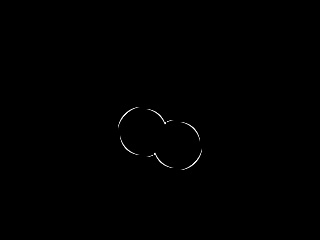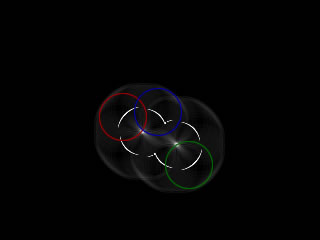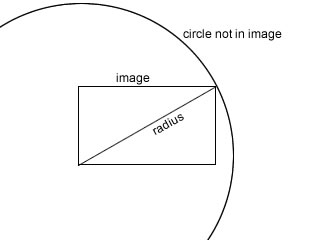Circle Hough Transform

Here I'll tell you how to detect circles (which are quite important in computer vision application) using a technique similar to the standard Hough transform. This article assumes you know how the Hough transform works, or you've understood the previous articles in this series (The Hough Transform).

The parameterization

A circle can be described completely with three pieces of information: the center (a, b) and the radius. (The center consists of two parts, hence a total of three)

x = a + Rcosθ

y = b + Rsinθ

When the θ varies from 0 to 360, a complete circle of radius R is generated.

So with the Circle Hough Transform, we expect to find triplets of (x, y, R) that are highly probably circles in the image. That is, we want to find three parameters. Thus, the parameter space is 3D... meaning things can get ugly if you don't tread slowly. Out of memory errors are common even if your programming language uses virtual memory.

So we'll start simple.

Assuming R is known

To begin, we'll start with the assumption that you're looking for circles of a particular radius, that is, R is known. The equation of each circle is:

x = a + Rcosθ

y = b + Rsinθ

So, every point in the xy space will be equivalent to a circle in the ab space (R isn't a parameter, we already know it). This is because on rearranging the equations, we get:

a = x1 - Rcosθ

b = y1 - Rsinθ

for a particular point (x1, y1). And θ sweeps from 0 to 360 degrees.

So, the flow of events is something like this:

2. Detect edges and generate a binary image
3. For every 'edge' pixel, generate a circle in the ab space
4. For every point on the circle in the ab space, cast 'votes' in the accumulator cells
5. The cells with greater number of votes are the centers

Here's an example:We'd like to find circles in this image. First, detect edges to get an image something like this:I used a sobel operator to get the images. And finally, for every white pixel in the above image, you create a circle in the ab-space. So, the ab space looks something like this:The horizontal axis is the 'a' axis, the vertical axis is the 'b' axis. The brighter a spot, more the number of votes case at the point. And more votes imply a greater probability of a point being a center.

In the above image, you can see the centers clearly. And these points can be easily extracted.

Here's a superimposed image that might help you understand the idea even better:In the above image, three random points were chose. Circles of radius R are drawn around them (the red, blue and green circles). And then, votes are cast at the pixels of these circles. Simple as that.

Note that the technique worked even though the entire circle's perimeter was not visible. The two circles overlapped, and yet they were detected as separate circles.

When R is not known

When the radius is not known, the simplest solution is to just guess. Assume R = 1, and then run the same algorithm. Then assume R = 2, and run it again. Assume R = 3.... and so on.

Whats the upper limit of R? A safe limit would be the length of the diagonal of the image. No possible circle on the image can have a radius greater than or equal to the diagonal.So, you'll end up with a 3D parameter space. Each horizontal plane would be equivalent to a 2D parameter space where R is known.

Also, you'll end up with a double cone around the centers. Here's an example. This of this like a CAT scan... going through the different slices of ab planes:

[flv:/static/img/tut/hough_circle.flv 320 240]

Some issues

Accuracy

The accuracy depends on the number of accumulator cells you have. If you have cells for 0, 0.01, 0.02, 0.03, and so on, you'll get better results than 0, 1, 2, 3. But at the same time, the amount of memory required increases.

Spurious circles

Sometimes, spurious circles are detected. This can happen if the image has a lot of circles. Close to each other. This problem can be overcome by just checking if a circle actually exists at the highest voted centers.

Related posts7 unique neural network architecturesEigenvectors

Here's a simple task - given an image find the dominant colors in the image. I'll walk you through a lesser known technique that does not use kmeans.

Utkarsh Sinha created AI Shack in 2010 and has since been working on computer vision and related fields. He is currently at Microsoft working on computer vision.